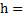# From a building two ballstoare thrown such thatis thrown upwards anddownwards (both vertically). Ifandare their respective velocities on reaching the ground, then a)b)c)d) Their velocities depends on their masses

## Question ID - 50940 :- From a building two ballstoare thrown such thatis thrown upwards anddownwards (both vertically). Ifandare their respective velocities on reaching the ground, then a)b)c)d) Their velocities depends on their masses

3537

 (b) From conservation of energy, Potential energy at heightkinetic energy at ground Therefore, at height, potential energy of ballPE =KE at groundSo,Similarly,Therefore,Next Question :

The average expenditure of a man for the first five months is Rs. 1200 and for the next seven months is Rs. 1300. If he saves Rs. 2900 in that year, his monthly average income is

(a) Rs. 1500                             (b) Rs. 1600                        (c) Rs. 1700                (d) Rs. 1400